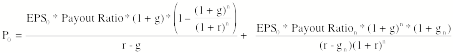Weekly Challenge 7

Using the equation for the two-stage dividend discount model, isolate the determinants of the PEGY ratio. The PEGY ratio is

PEGY = PE/ (Expected growth rate + Dividend Yield)

Dividend yield = DPS0/ P0

The equation for the two-stage dividend discount model is reproduced below:Using the equation that you end up with for the PEGY ratio estimate the PEGY ratio for a hypothetical firm (make up a growth rate, payout ratio and beta) and construct a perfect under valued firm.